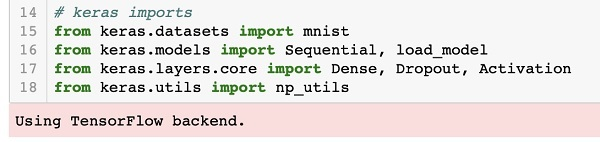# Deep Learning with Keras - Importing Libraries

We first import the various libraries required by the code in our project.

## Array Handling and Plotting

As typical, we use numpy for array handling and matplotlib for plotting. These libraries are imported in our project using the following import statements

```import numpy as np
import matplotlib
import matplotlib.pyplot as plot
```

## Suppressing Warnings

As both Tensorflow and Keras keep on revising, if you do not sync their appropriate versions in the project, at runtime you would see plenty of warning errors. As they distract your attention from learning, we shall be suppressing all the warnings in this project. This is done with the following lines of code −

```# silent all warnings
import os
os.environ['TF_CPP_MIN_LOG_LEVEL']='3'
import warnings
warnings.filterwarnings('ignore')
from tensorflow.python.util import deprecation
deprecation._PRINT_DEPRECATION_WARNINGS = False
```

## Keras

We use Keras libraries to import dataset. We will use the mnist dataset for handwritten digits. We import the required package using the following statement

```from keras.datasets import mnist
```

We will be defining our deep learning neural network using Keras packages. We import the Sequential, Dense, Dropout and Activation packages for defining the network architecture. We use load_model package for saving and retrieving our model. We also use np_utils for a few utilities that we need in our project. These imports are done with the following program statements −

```from keras.models import Sequential, load_model
from keras.layers.core import Dense, Dropout, Activation
from keras.utils import np_utils
```

When you run this code, you will see a message on the console that says that Keras uses TensorFlow at the backend. The screenshot at this stage is shown here −Now, as we have all the imports required by our project, we will proceed to define the architecture for our Deep Learning network.